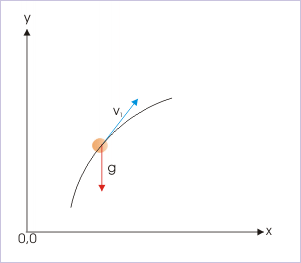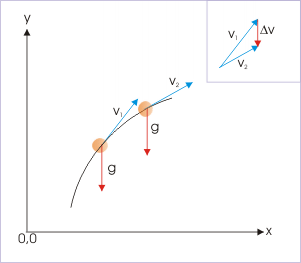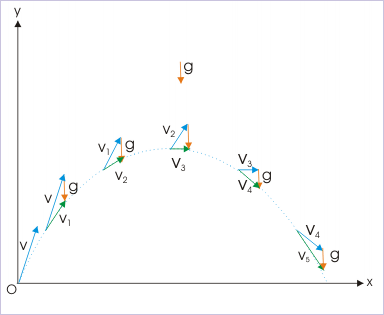# 2.4 Constant acceleration  (Page 2/4)

 Page 2 / 4

The important fall out of a constant acceleration is that its magnitude has a constant value and its direction is fixed. A change in either of the two attributes, constituting acceleration, shall render acceleration variable. This means that acceleration is along a straight line. But does this linear nature of acceleration mean that the associated motion is also linear? Answer is no.

Reason is again the “disconnect” between acceleration and velocity. We know that magnitude and direction of acceleration are solely determined by the mass of the object and net external force applied on it. Thus, a constant acceleration only indicates that the force i.e the cause that induces change in motion is linear. It does not impose any restriction on velocity to be linear.Force and velocity are in two different directions

It is imperative that if the initial velocity of the object is not aligned with linear constant acceleration like in the figure above, then the immediate effect of the applied force, causing acceleration, is to change the velocity. Since acceleration is defined as the time rate of change in velocity, the resulting velocity would be so directed and its magnitude so moderated that the change in velocity (not the resulting velocity itself) is aligned in the direction of force.The change in velocity has the same direction as that of acceleration.

As the resulting velocity may not be aligned with the direction of force (acceleration), the resulting motion may not be linear either. For motion being linear, it is essential that the initial velocity and the force applied (and the resulting acceleration) are aligned along a straight line.

Examples of motions in more than one dimension with constant acceleration abound in nature. We have already seen that motion of a projectile in vertical plane has constant acceleration due to gravity, having constant magnitude, g, and fixed downward direction. If we neglect air resistance, we can assume that all non- propelled projectile motions above ground are accelerated with constant acceleration. In the nutshell, we can say that constant acceleration is unidirectional and linear, but the resulting velocity may not be linear. Let us apply this understanding to the motion of a projectile, which is essentially a motion under constant acceleration due to gravity.The velocity of the ball is moderated by acceleration.

In the figure, see qualitatively, how the initial velocity vector, v, is modified by the constant acceleration vector, g, at the end of successive seconds. Note that combined change in both magnitude and direction of the velocity is taking place at a constant rate and is in vertically downward direction.

In the context of constant acceleration, we must also emphasize that both magnitude and direction are constant. A constant acceleration in magnitude only is not sufficient. For constant acceleration, the direction of acceleration should also be same (i.e constant). We can have a look at a uniform circular motion in horizontal plane, which follows a horizontal circular path with a constant speed.

#### Questions & Answers

What are the system of units
Jonah Reply
A stone propelled from a catapult with a speed of 50ms-1 attains a height of 100m. Calculate the time of flight, calculate the angle of projection, calculate the range attained
Samson Reply
58asagravitasnal firce
Amar
water boil at 100 and why
isaac Reply
what is upper limit of speed
Riya Reply
what temperature is 0 k
Riya
0k is the lower limit of the themordynamic scale which is equalt to -273 In celcius scale
Mustapha
How MKS system is the subset of SI system?
Clash Reply
which colour has the shortest wavelength in the white light spectrum
Mustapha Reply
how do we add
Jennifer Reply
if x=a-b, a=5.8cm b=3.22 cm find percentage error in x
Abhyanshu Reply
x=5.8-3.22 x=2.58
sajjad
what is the definition of resolution of forces
Atinuke Reply
what is energy?
James Reply
Ability of doing work is called energy energy neither be create nor destryoed but change in one form to an other form
Abdul
motion
Mustapha
highlights of atomic physics
Benjamin
can anyone tell who founded equations of motion !?
Ztechy Reply
n=a+b/T² find the linear express
Donsmart Reply
أوك
عباس
Quiklyyy
Sultan Reply
Moment of inertia of a bar in terms of perpendicular axis theorem
Sultan Reply
How should i know when to add/subtract the velocities and when to use the Pythagoras theorem?
Yara Reply

### Read also:

#### Get the best Physics for k-12 course in your pocket!

Source:  OpenStax, Physics for k-12. OpenStax CNX. Sep 07, 2009 Download for free at http://cnx.org/content/col10322/1.175
Google Play and the Google Play logo are trademarks of Google Inc.

Notification Switch

Would you like to follow the 'Physics for k-12' conversation and receive update notifications?ByByBy